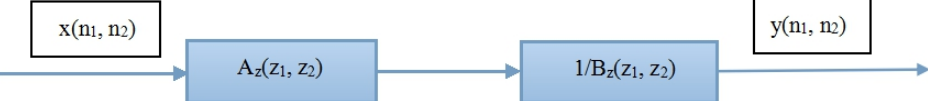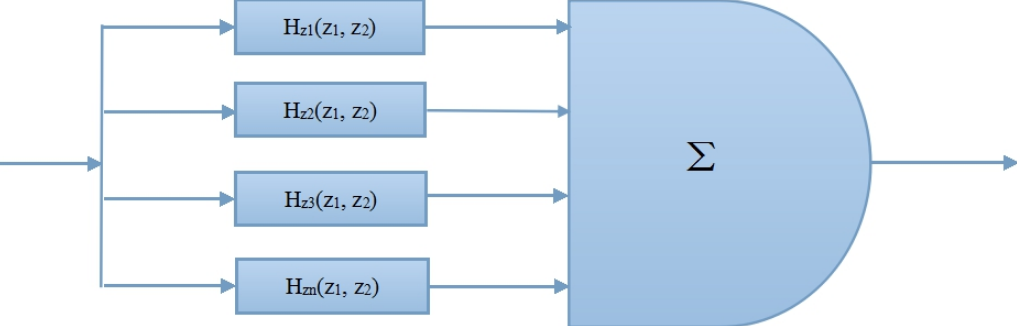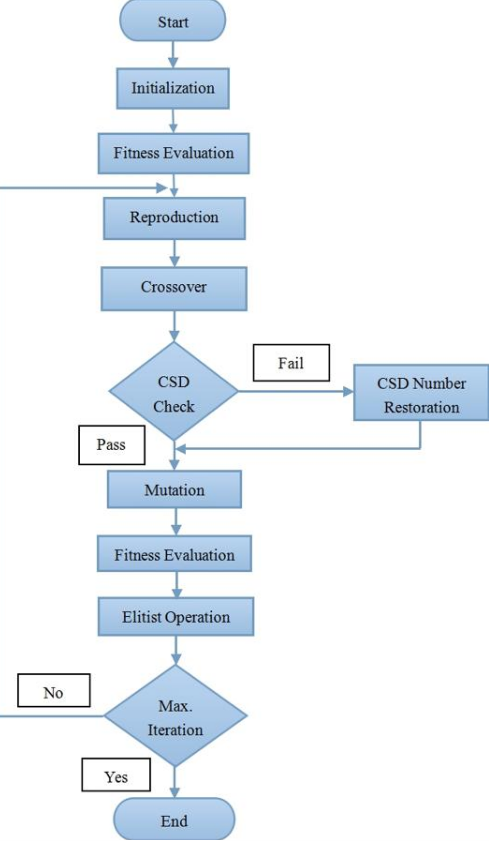Submitted Successfully!
Thank you for your contribution! You can also upload a video entry or images related to this topic.
Ver. Summary Created by Modification Content Size Created at Operation
1 handwiki -- 1468 2022-10-26 01:50:08

Do you have a full video?

# Confirm

Are you sure to Delete?
Cite
Xu, H. 2D Filters. Encyclopedia. Available online: https://encyclopedia.pub/entry/31414 (accessed on 01 December 2023).
Xu H. 2D Filters. Encyclopedia. Available at: https://encyclopedia.pub/entry/31414. Accessed December 01, 2023.
Xu, Handwiki. "2D Filters" Encyclopedia, https://encyclopedia.pub/entry/31414 (accessed December 01, 2023).
Xu, H.(2022, October 26). 2D Filters. In Encyclopedia. https://encyclopedia.pub/entry/31414
Xu, Handwiki. "2D Filters." Encyclopedia. Web. 26 October, 2022.
2D Filters

Two dimensional filters have seen substantial development effort due to their importance and high applicability across several domains. In the 2-D case the situation is quite different from the 1-D case, because the multi-dimensional polynomials cannot in general be factored. This means that an arbitrary transfer function cannot generally be manipulated into a form required by a particular implementation. The input-output relationship of a 2-D IIR filter obeys a constant-coefficient linear partial difference equation from which the value of an output sample can be computed using the input samples and previously computed output samples. Because the values of the output samples are fed back, the 2-D filter, like its 1-D counterpart, can be unstable.

multi-dimensional applicability transfer function

## 1. Motivation & Applications

Due to the rapid development of information science and computing technology, the theory of digital filters design and application has achieved leap-forward development. We encounter a variety of signals in real-life, such as broadcasting signals, television signals, radar signals, mobile phone signals, navigation signals, radio astronomy signal, biomedical signals, control signals, weather signal, seismic signal, mechanical vibration signal, remote sensing and telemetry signals, etc. Most of these signals are analog signals and just a small portion of them are digital signals. The analog signals are continuous function of the independent variables, which can be one-dimensional, two-dimensional or multidimensional. In most cases, the variable of one-dimensional analog signals are time. After the time sampling and discretization of magnitude, such analog signal will become a one-dimensional digital signal. The resulting digital signal can be represented by a discrete sequence. For example, one common signal is voice signal. An example of a two-dimensional signal is an image. A filter is a system that can transform a signal into another signal. Examples of such transformation include smoothing the signal for noise removal, removing frequency components from a signal and amplifying frequency components for signal enhancement. The design and implementation of filter is an important branch in signal analysis and processing technology. Filters also play a main role in signal acquisition, transmission, processing and exchange.

## 2. Problem Statement & Basic Concepts

### 2.1. Digital Filters

Digital signal filtering is implementing a digital filter. A digital filter is a system that performs mathematical operations on a sampled, discrete-time signal to reduce or enhance certain aspects of that signal. The input and output signals are all digital signals. This is in contrast to the other major type of electronic filter, the analog filter, which is an electronic circuit operating on a continuous-time analog signal. Actually the basic concept of digital filters and analog filters are the same. The only difference is the types of signals and the methods to filtering. Digital filters can be implemented numerically in software and have the advantages of high processing accuracy, steady system, little volume and light weight. There is no impedance matching in digital filters and digital filters can accomplish some special filtering functions that can’t be accomplished by analog filters. Analog signals can also be processed through digital filters by using Analog to Digital converters.

### 2.2. Two-Dimensional Digital Filters

Two-dimensional filters are used to process two-dimensional digital signals. There is an important difference between the design of 1-D and 2-D digital filter problems. In 1-D case, the design and the implementation of filters can be more easily considered separately. The filter can first be designed and then, through the appropriate manipulations of the transfer function, the coefficients required by a particular network structure can be determined. While in the 2-D case, the design and implementation are more closely related. Since multidimensional polynomials can’t be factored in general. This means that an arbitrary multi-dimensional transfer function can generally not be manipulated into a form required by a particular implementation. If our implementation can realize only factorable transfer functions, our design algorithm must be tailored to design only filters of this class. This has the effect of complicating the design problem and also limiting the number of practical implementations. Digital filters can be categorized into two main types, namely finite impulse response (FIR) and infinite impulse response (IIR). 2-D FIR digital filter is achieved by a non-recursive algorithm structure while 2-D IIR digital filter is achieved by a recursive feedback algorithm structure.

## 3. Existing Approaches

### 3.1. Direct Form Implementations of 2-D IIR Filters

An IIR filter may be implemented in direct form by rearranging its difference equation to express one output sample in terms of the input samples and previously computed output samples. For a first-quadrant filter, the input signal $\displaystyle{ x\left(n_1,n_2\right) }$ and the output signal $\displaystyle{ y\left(n_1,n_2\right) }$ are related by

$\displaystyle{ y\left(n_1,n_2\right)=\sum_{l_1=0}^{L_1-1}\sum_{l_2=0}^{L_2-1}a(l_1,l_2)x(n_1-l_1,n_2-l_2)-\sum_{k_1=0}^{K_1-1}\sum_{k_2=0}^{K_2-1}b(k_1,k_2)y(n_1-k_1,n_2-k_2) }$

Since the response of the filter to an impulse $\displaystyle{ \delta(n_1,n_2) }$ is by definition the impulse response $\displaystyle{ h\left(n_1,n_2\right) }$, we can derive the relationship

$\displaystyle{ h\left(n_1,n_2\right)=a(l_1,l_2)-\sum_{k_1=0}^{K_1-1}\sum_{k_2=0}^{K_2-1}b(k_1,k_2)h(n_1-k_1,n_2-k_2) }$

By taking the 2-D z-transform of both sides, we can solve for the system function $\displaystyle{ H\left(z_1,z_2\right) }$, which is given by

$\displaystyle{ H_z(z_1,z_2)=\frac{\sum_{l_1=0}^{L_1-1}\sum_{l_2=0}^{L_2-1}a(l_1,l_2)z_1^{-l_1}z_2^{-l_2}}{\sum_{k_1=0}^{K_1-1}\sum_{k_2=0}^{K_2-1}b(k_1,k_2)z_1^{-k_1}z_2^{-k_2}}=\frac{A_z(z_1,z_2)}{B_z(z_1,z_2)} }$

This ratio may be viewed as resulting from the cascade of two filters, an FIR filter with a system function equal to$\displaystyle{ A(z_1,z_2) }$ and an IIR filter with a system function equal to $\displaystyle{ 1/B(z_1,z_2) }$, as shown in the figure below.Representation for the filter with the system function $\displaystyle{ H(z_1,z_2) }$. Adapted from .

### 3.2. Parallel Implementations of 2-D IIR Filters

Another method to build up complicated 2-D IIR filters is by the parallel interconnection of subfilters. In this case, the overall transfer function becomes

$\displaystyle{ H_z^p(z_1,z_2)=\sum_{i=1}^NH_z^{(i)}(z_1,z_2) }$

Using equation

$\displaystyle{ H_z^{(i)}(z_1,z_2)=\frac{A_z^{(i)}(z_1,z_2)}{B_z^{(i)}(z_1,z_2)} }$

and putting the sum in transfer function over a common denominator, we get the expanded form

$\displaystyle{ H_z^{p}(z_1,z_2)=\frac{A_z^{p}(z_1,z_2)}{B_z^{p}(z_1,z_2)}=\frac{\sum_{j=1}^N\prod_{i j}A_z^{(j)}(z_1,z_2)B_z^{(j)}(z_1,z_2)}{\prod_{i=1}^NB_z^{(i)}(z_1,z_2)} }$

The parallel form cannot be used to implement an arbitrary 2-D rational system function. Nevertheless, we can synthesize interesting 2-D IIR filters which can be implemented by a parallel architecture. For example, the parallel form may be advantageous when designing a filter with multiple passband. The parallel implementation can also be useful for implementing a 2-D IIR filter whose impulse response is not confined to a single quadrant, such as a symmetric filter.Parallel interconnection of N simple 2-D IIR filters to form a new 2-D IIR filter. Adapted from .

### 3.3. Design of 2-D IIR Filters with Genetic Algorithm

Many design techniques for 2-D IIR digital filters have been reported in the literature(). During the past decade, genetic algorithm has been successfully used to digital filter design. Here we present a method for designing 2-D IIR Filters called GA-Based design method.

#### Initialization

The figure below shows the proposed GA-Based design flow. Filter coefficients are encoded in their CSD number representation. In the population initialization, chromosomes are generated randomly. Each coefficient has the pre-specified wordlength and maximum number of non-zero digits, which can be set to any desired values.GA-based Design Flow chart. Adapted from .

#### Genetic Operators

Roulette Wheel Selection is used as the reproduction operator. After each crossover operation, the coefficient where the crossover point lies in will be checked upon CSD format. Mutation operation is the simple single bit flip. After mutation, each coefficient in the offspring is checked upon CSD format.

#### Fitness Evaluation and Replacement Strategy

The fitness evaluation is a two-step process. The first step is to check the stability of each second order section using the stability triangle. Chromosomes failing the check are assigned fitness value 0. Elitist strategy is applied for old generation replacement. After reproduction the best chromosome and the worst chromosome in the offspring are found out. The designed filter has non-separable numerator and separable denominator transfer function. The number restoration technique is used to ensure that the filter coefficients are represented in the pre-specified CSD format.

### References

1. T. S. Huang, “Stability of two-dimensional recursive filters,” IEEE Transactions on Audio and Electroacoustics, vol. 20, no. 2, pp. 158–163, 1972.
2. J. S. Lim, Two-Dimensional Signal and Image Processing, Prentice-Hall International, 1990.
3. Dan E. Dudgeon, Russell M. Mersereau, “Multidimensional Digital Signal Processing”, Prentice-Hall Signal Processing Series, ISBN:0136049591, 1983.
4. M. Ahmadi, “Design of 2-Dimensional recursive digital filters”, Control and Dynamics System, vol. 78, pp. 131-181, 1996.
5. Li Liang, Majid Ahmadi, Maher Sid-Ahmed, “Design of 2-D IIR FIlters with Canonical signed-digit coefficients using genetic algorithm”, Department of Electrical & Computer Engineering, University of Windsor, Canada.
6. A. Mazinani, M. Ahmadi, M. Shridhar and R. S. Lashkari, “A novel approach to the design of 2-D recursive digital filters”, Journal of the Franklin Institute, Pergamon Press Ltd, vol. 329, no. 1, pp. 127-133, 1992.
More
Information
Subjects:
Contributor MDPI registered users' name will be linked to their SciProfiles pages. To register with us, please refer to https://encyclopedia.pub/register :
View Times: 422
Entry Collection:
Revision: 1 time (View History)
Update Date: 26 Oct 2022
1000/1000